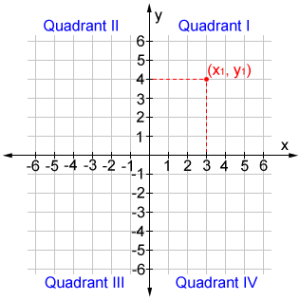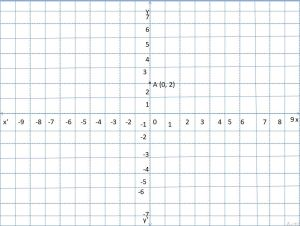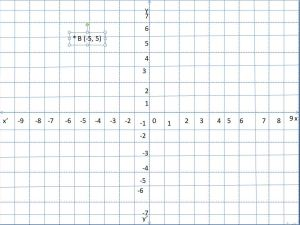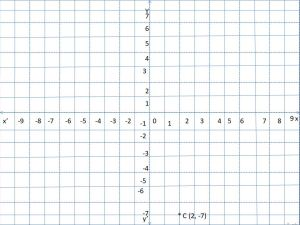# Coordinate Graph Definition | Plot Ordered Pair on Coordinate Graph

A coordinate graph is a two-dimensional plane having two perpendicular lines intersect at the midpoint called the origin. It is also called the cartesian plane or coordinate plane. You can represent ordered points in the coordinate graph. It is needed for the students who are studying the class 8 coordinate geometry concept. So, students are advised to go through this entire article to get clarity on what is meant by the coordinate graph, why it is used, solved examples, and how to represent an ordered pair in the coordinate graph.

## Coordinate Graph Definition

A coordinate graph has two lines that are perpendicular to each other. Those lines are called axes. The horizontal line is known as the x-axis, and the vertical line is known as the y-axis. Those two axes meet at zero. The point o intersection of axes is called the origin. This coordinate graph is also called the coordinate plane or cartesian plane or cartesian coordinate system.

### What is meant by Coordinates Axes, Quadrants?

On a coordinate graph two perpendicular straight lines having names X’OX, Y’OY are called the coordinate axes. The line having the name X’OX is called the x-axis, the other line having the name Y’OY is called the y-axis, and the point O is called the origin. Each graph paper has both coordinate axes (x-axis, y-axis) is known as the cartesian plane.• The first quadrant, is a quadrant having both x and y coordinates positive. The plane having xoy is called quadrant 1.
• The second quadrant is available to the left side of the first quadrant, it has abscissa as negative and ordinate as positive. The plane enclosed by yox’ is quadrant 2.
• The third quadrant is available below the second quadrant and here both abscissa, ordinates are negative. The plane enclosed by x’oy’ is called quadrant 3.
• The last quadrant or fourth quadrant is available right-hand side to the third quadrant and below the first quadrant. So, the x coordinate value is positive and the y coordinate value is negative.

### Steps to Plot Ordered Pairs on a Coordinate Graph

The ordered pair is a point in the coordinate plane having both x-coordinate and y-coordinate values. It has two real numbers enclosed by braces ‘(‘, ‘)’ and separated by a comma. The first value in the ordered pair is called the abscissa or x coordinate and the second value is called the y coordinate or ordinate. The format of an ordered pair is (x_coordinate_value, y_coordinate_value). In the following sections, we are giving the simple steps and instructions to mark ordered pairs on a coordinate graph effortlessly. Have a look at them and follow them whenever necessary.

• Let us take any ordered pair having 2 real numbers as abscissa and ordinate.
• Check out the signs of those real numbers to determine under which quadrant the given point lies.
• Then take the mentioned number of units on the x-axis and y-axis.
• Highlight that point and write the ordered pair there.
• Similarly, you can plot several ordered pairs.
• And join those ordered pairs to get a shape.

### Example Questions

Question 1.

Plot point A (0, 2) on the graph?

Solution:

Given ordered pair is A (0, 2)

The coordinates of the point are positive. So, the point lies in the first quadrant.

As the x coordinate value is zero, the point lies on the y-axis.

As the y coordinate is 2, the point is 2 units away from the y-axis.Question 2.

Plot point B (-5, 5) on the graph?

Solution:

Given ordered pair is B (-5, 5)

The x coordinate of B is negative and the y coordinate is positive.

According to the coordinate graph rules, the point belongs to the second quadrant.

Take -5 units on the x-axis and 5 units on the y-axis to get point B.Question 3.

Plot point C (2, -7) on the graph?

Solution:

Given ordered pair is C (2, -7)

As the abscissa of the point is positive and the ordinate is negative, the ordered pair lies in the fourth quadrant.

Measure 2 units on the x-axis and move to the ordinate part. Measure -7 units on the y-axis. Mark that point as the ordered pair C (2, -7).### Frequently Asked Questions on Coordinate Plane

1. What are the 4 parts of a coordinate plane?

The major four different parts of a coordinate plane are the first quadrant, second quadrant, third quadrant, and fourth quadrant. These quadrants are obtained by drawing two straight and perpendicular lines on the coordinate graph. These points meet one other at the origin. All these quadrants are adjacent and the 1st, 2nd is above the 3rd and 4th quadrants. Based on the sign of coordinates of a point, we can say the point stays on which quadrant.

2. What is a coordinate plane example?

We have so many real-time examples of a cartesian plane. One of them is when you are planning to arrange furniture in a room divide the room into four parts and place the object at a place and know the area occupied by that particular object in the room. Later, decide based on your wishes and requirements.

3. What are the coordinates on a graph?

An ordered pair (a, b) gives the position of a point on a coordinate plane where a is the number on the x-axis and b is the number on the y-axis. The values of a, b in the ordered pair are called the coordinates on a graph.